français | español | polski | 日本語 |

Pokemon Sinapore Math 3B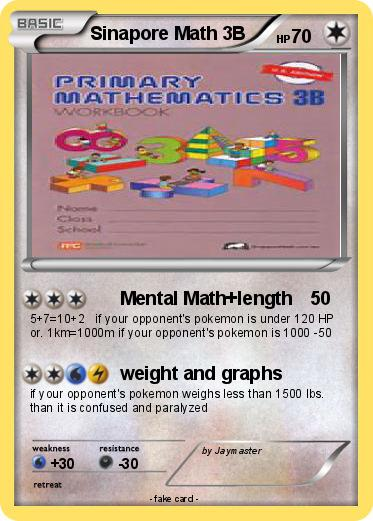Language Card : english

Creation Date : 28 January 2015

### Pokemon Passport

Name : Sinapore Math 3B

Serie : Black & White

Type : Colorless

Attack 1 : Mental Math+length
5+7=10+2 if your opponent's pokemon is under 120 HP or. 1km=1000m if your opponent's pokemon is 1000 -50

Attack 2 : weight and graphs
if your opponent's pokemon weighs less than 1500 lbs. than it is confused and paralyzed

Comments : by Jaymaster

### Vote for this card

(3 votes)

Related cards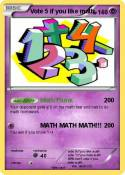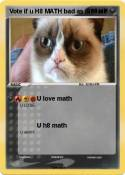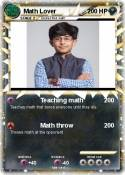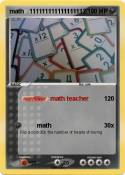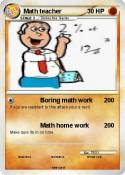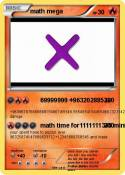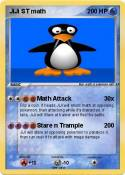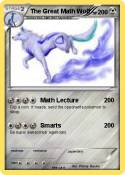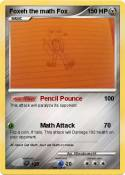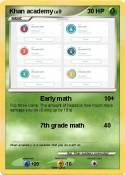Vote 5 if you hate math | Math Mann | I Can Do Math Everyday! | MATH | Math Teacher | MATh | math team | Math Book | Math knight | Brice | Math | Math Teacher | Math :( | math 100 x | me in math | Math man | Daniel EX | Nerd | math | Math Homework | Cool Math | math doesn't lie | Tushar | Math 24 | Math Knight | Math Man | Math | Boring Math Teacher | Math | Mrs Ferriola | 1+1 | Ratey hte math cat | St Math | Mrs Baker | Math | Math | Math | Math | more math | math | Asian guy | Asian guy | School | Math | Math Quiz | Vote if you like math | Matt's math degree | ??????????????? | njo | Math | Pi | ??? | math | PIE | can you Math????? | math pig | connor | math | M I C H A L | Homework | Mr pop | Nerdy | F-Man | = | Math | math | Bob | LEAFATARR | Kid | God Damn

Comments

Pokémon is a registered trademark of Nintendo, Creatures, Game Freak and The Pokémon Company
MyPoKeCaRd.cOm 0.9 - Propulsed by jquery, php, gd and spip - XHTML 1 and CSS2 valid page
MyPokeCard.com is a funny site to design your own pokemon card, vote for the best pokemon cards and create pokemon colorings